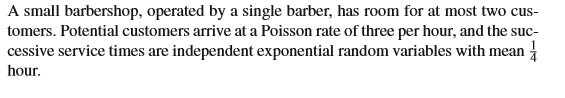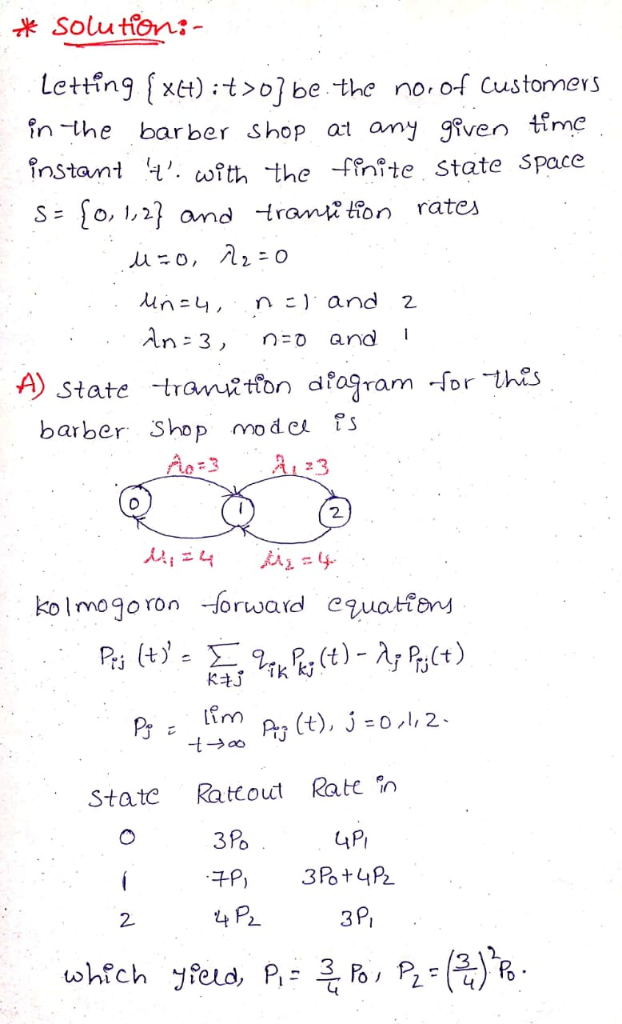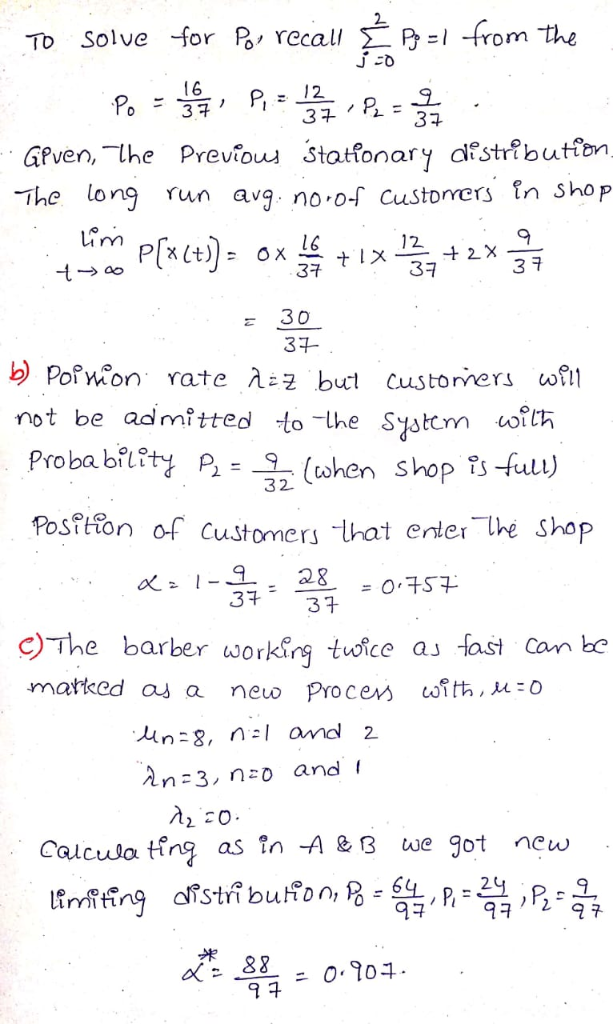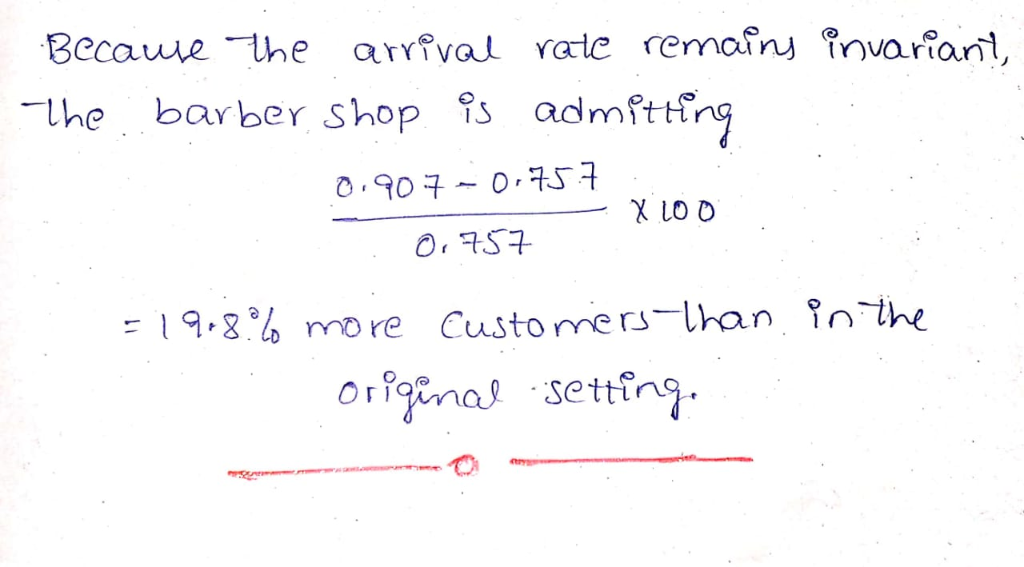# Create the transition rate diagram and the probability matrix for this problem.Create the transition rate diagram and the probability matrix for this problem.

A small barbershop, operated by a single barber, has room for at most two cus- tomers. Potential customers arrive at a Poisson rate of three per hour, and the suc- cessive service times are independent exponential random variables with mean hour##### Add Answer of: Create the transition rate diagram and the probability matrix for this problem.
More Homework Help Questions Additional questions in this topic.

• #### Consider a DTMC X;n 2 0 with state space E 0,1,2,... ,N), and transition probability matrix P = (...

Need Online Homework Help?